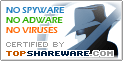# RP Measure Converter 3

SNAPSHOT

powerfull unit conversion add in for excel

Windows AllPlatform :
USD \$7Price :
227 KBFile Size :
ScreenshotScreenshot :Popularity :Measure conversion add-in for Excel. This add-in can convert Length, Mass, Temperature, Time, Area, Volume, Strength, Pressure, Power, viscosity and ANY combination of these measures (speed, density, etc).

The function are exposed as user defined functions and can be used as Excel formulas.

Main features compared to ordinary conversion tools:
i) you can convert unit combinations using multiplication (dot sign) and division (/ sign) operations
2 meters per hour to centimeters per second: unit(2,"m/h","cm/s")
3 pound-force times feet to Joule: unit(3,"lbf.ft","J")
ii) you can check unit conversion consistency
unit(3,"lbf.ft","J") gives you the error message: [lbf]=M^1 L^1 T^2 and [J]= M^1 L^2 T^-2
iii) you can use International System or usual multipliers
MJ stands for Mega Joule and M J stands for thousand Joules
cP stands for centipoise
iv) you can use ( ) and
v) you can use the features alltogether